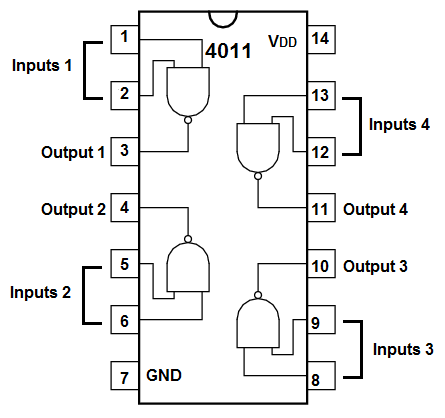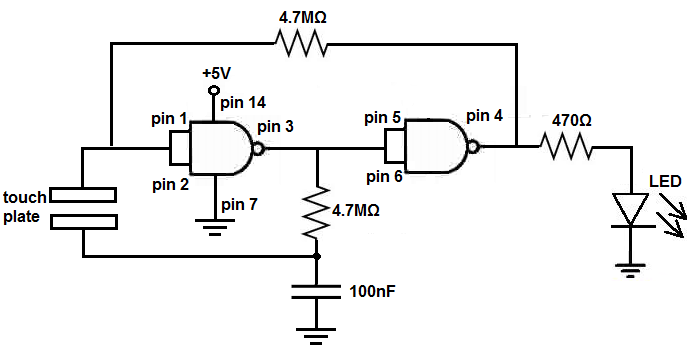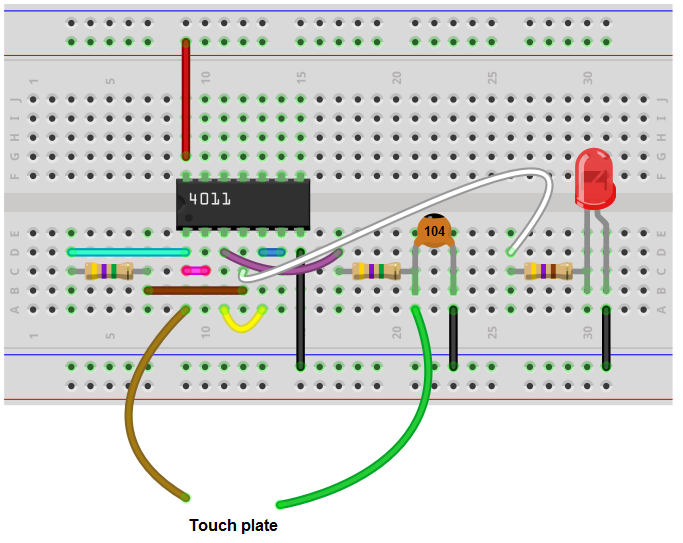﻿ How to Build a Touch On-Off Circuit with a 4011 NAND Gate Chip ﻿# How to Build a Touch On-Off Circuit with a 4011 NAND Gate ChipIn this circuit, we will build a touch on-off switch circuit using a 4011 NAND gate chip.

The NAND gate chip circuit will function as a flip flop.

When we touch the touch wire, the LED turns on. And if we touch it again, the LED turns off.

This circuit truly functions as an on-off touch sensor circuit.

This is the same type of circuit we would see in a touch lamp, where you touch it on or off.

The main reason how this circuit can do this is through the use of a capacitor. In this circuit, we use a 100nF ceramic capacitor. When we touch the wire, we either charge up the capacitor or discharge the capacitor. Charging it up turns the LED off. And discharging the capacitor turns the LED on. We'll go over this in much more depth below.

We exploit NAND gate logic in order to make this circuit possible.

In this circuit, our output device will be an LED, which will turn on and off, as we touch the touch plate or touch wire.

Components

• 4011 NAND Gate Chip
• 2 4.7MΩ resistors
• 470Ω resistor
• 100nF ceramic capacitor
• Touch Plate

The 4011 quad NAND gate chip can be obtained very cheaply from a number of online retailers for just a few cents. One place it can be obtained from is Tayda Electronics at the following link: Tayda Electronics- 4011 Quad 2-Input NAND Gate IC. However, it is a very popular chip and many electronics parts suppliers have them.

The pinout of the 4011 is shown below, so that you can see how to connect it in the circuit.Each NAND gate has input pins and 1 output pin.

The following chart shows NAND gate logic, which shows what output a NAND gate chip will produce for a set of given inputs.

 NAND Gate Logic Inputs Output 0 0 1 0 1 1 1 0 1 1 1 0

This means that if one of the inputs are a 0, the NAND gate will output a logic HIGH at its output, which means the output will be drawn up to VCC and the load will be powered. If both inputs feeding into the NAND gate are a 1, only then will the NAND gate output a logic LOW at its output, which means the output will be drawn down to GND, and the load will not be powered.

This will use this NAND logic principle to build our circuit.

### Touch On-Off Circuit Using a 4011 NAND Gate Chip

The touch on-off circuit we will build with a 4011 NAND gate chip is shown below.To see this circuit diagram connection from the view of the integrated circuit (IC), see the following: Touch On-Off Circuit with a 4011 NAND Gate Chip shown from the IC

The breadboard schematic of the circuit above is shown below.First, we must connect power to the 4011 NAND gate chip. We do this by by connecting VCC, pin 14, to 5V and by connecting GND, pin 7, to power ground. This establishes power to the 4011 NAND gate chip.

Pins 1 and 2 are tied common.

Pins 5 and 6 are tied common.

The output of the first gate is connected to the input of the second gate. So pin 3 is connected to pins 5 and 6.

A 4.7MΩ resistor connects across pins 1 and 4.

We then connect a 4.7MΩ resistor and a 100nF capacitor to pin 3, the output of the first gate. Since the output of the first gate is connected to the input of the second gate, the resistor and capacitor are in between the 2 gates.

We connect a plate or wires to the pin 1 and in between the 4.7MΩ resistor and the 100nF capacitor. When touched, these wires allow for the output to switch either from HIGH to LOW or LOW to HIGH.

Lastly, we connect the output device that we want to turn on and off to pin 4, which is the output of the second gate, which is the total output of the circuit, since we're using 2 gates. The LED has a 470Ω current-limiting resistor to prevent the LED from blowing out due to excess current.

This completes all the hardware connections.

### How the Circuit Works

This circuit is somewhat complex but still follows the simple principle of NAND gate logic. When the inputs are LOW, the output will be HIGH. So when the inputs to the NAND gate are LOW, our output device, the LED, will be ON. When the 2 inputs are HIGH, the output will be LOW. So, in this case, the LED will be off.

NAND gate logic is what this circuit follows. It's on when the inputs to the first gate are LOW. And it's off when the inputs to the first gate are HIGH.

We tie the input pins on both NAND gates together for this simple reason. If both are tied together, they both contain the same value, since they're common. So if 1 is HIGH, they're both HIGH. If 1 is LOW, they're both LOW. This simplifies the possibilities. They're either going to be both HIGH or both LOW. When they're both LOW, the output is on. When they're both HIGH, the output is off.

The heart of our circuit which allows the flip flop ability is the 4.7MΩ resistor in series with the 100nF capacitor.

When we touch the touch plate, we either charge up the capacitor; it charges to a voltage very close to the value of VCC. Or we discharge the capacitor; it discharges to near 0V.

When we charge up the capacitor, we charge up to near VCC. With the 4.7MΩ resistor, it pulls up the output of the output of gate 1 to VCC. This pulls the value of the output HIGH. Since the output of gate 1 is connected to the input of gate 2, this means that gate 2 receives a HIGH input. This makes the output LOW. Therefore, the LED will be off when the capacitor is charged.

Now when we touch the touch plate again, we discharge the capacitor. The capacitor's voltage plummets from near VCC almost to 0V. Now the output of gate 1 is 0V or LOW. This LOW signal is now fed into the input of gate 2, which will turn on the output, the LED.

And this is how this circuit works to mimic a flip flop.

Related Resources